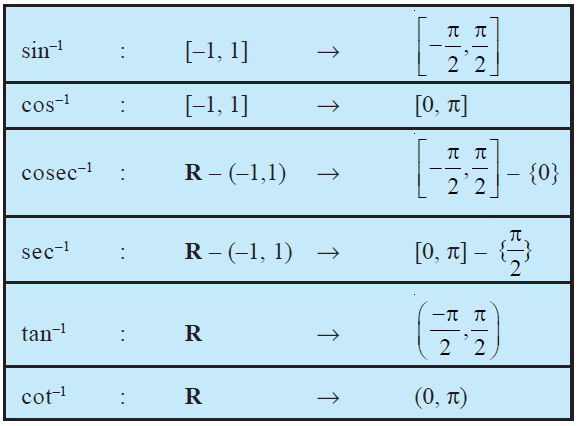Sunday, September 22, 2019
Home > Latest Announcement > NCERT Solutions For CBSE Maths Chapter 2 Inverse Trigonometric Functions

# NCERT Solutions For CBSE Maths Chapter 2 Inverse Trigonometric Functions

The inverse of a function f, denoted by f –1, exists if f is one-one and onto.

The domain and range of the trigonometric function are sine : R→ [– 1, 1], cos : R → [– 1, 1], tan : R – { x : x = (2n + 1)(π/2), n ∈ Z} → R, cot : R – { x : x = nπ, n ∈ Z} → R, sec : R – { x : x = (2n + 1)(π/2), n ∈ Z} → R – (– 1, 1), cosec : R – { x : x = nπ, n ∈ Z} → R – (– 1, 1).

If f : X→Y such that f (x) = y is one-one and onto, then we can define a unique function g : Y→X such that g(y) = x, where x ∈ X and y = f (x), y ∈ Y. Here, the domain of g = range of f and the range of g = domain of f. The function g is called the inverse of f and is denoted by f –1. Further, g is also one-one and onto and inverse of g is f. Thus, g–1 = (f –1)–1 = f. Thus, we have (f –1 o f ) (x) = f –1 (f (x)) = f –1(y) = x and (f o f –1) (y) = f (f –1(y)) = f (x) = y.

The following table gives the inverse trigonometric function (principal value branches) along with their domains and ranges.If no branch of an inverse trigonometric functions is mentioned, we mean the principal value branch of that function.

The value of an inverse trigonometric functions which lies in the range of principal branch is called the principal value of that inverse trigonometric functions.

If y = sin–1x, then x = sin y and if x = sin y, then y = sin–1x. This is equivalent to sin (sin–1 x) = x, x ∈ [– 1, 1] and sin–1 (sin x) = x, x ∈ [-π/2, π/2]. Same is true for other five inverse trigonometric functions as well.

error: Content is protected !!